### Vol 17, No 12 (2017) / Yang

Determination of the free lunar libration modes from ephemeris DE430

# Determination of the free lunar libration modes from ephemeris DE430

Yang Yong-Zhang1, 2, 3, , Li Jin-Ling1, 3, Ping Jin-Song2, 3, Hanada Hideo4

Shanghai Astronomical Observatory, Chinese Academy of Sciences, Shanghai 200030, China
National Astronomical Observatories, Chinese Academy of Sciences, Beijing 100012, China
University of Chinese Academy of Sciences, Beijing 100049, China
RISE Project, National Astronomical Observatory of Japan, Oshu 0230861, Japan

† Corresponding author. E-mail: yzyang@shao.ac.cn

Abstract: Abstract

The Moon’s physical librations have been extensively studied, and elaborate researches have been developed for the purpose of deriving accurate modes of free librations. Our motivation comes from the Planetary and Lunar Ephemeris DE430 by JPL/NASA, which was created in April 2013, and is reported to be the most accurate lunar ephemeris today using the data from Gravity Recovery and Interior Laboratory (GRAIL). Therefore, the residuals after fitting the model have reduced owing to improvement in the libration models, and the free librations embedded in the Euler angles have also improved. We use Fourier analysis to extract the approximate frequencies from DE430 and then a quadratic interpolation method is used to determine higher accuracy frequencies. With the frequencies, the linear least-squares fitting method is employed to fit the lunar physical librations to DE430. From this analysis we identified the three modes of free physical librations, and estimated the amplitudes as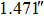in longitude,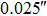in latitude and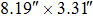for the wobble, with the respective periods of 1056.16, 8806.9 and 27262.99 d. Since the free librations damp with time, they require recent excitation or a continuous stimulating mechanism in order to sustain.

Keywords: Moon;physical librations;Fourier analysis;least squares method

## 1 Introduction

There are many moons which keep facing one side of their central planet on average in solar system bodies, including Earth’s Moon, most regular satellites of the giant planets and even Pluto’s moon Charon. This is called synchronous rotation, which was originally described in work by Cassini in the eighteenth century. For the Moon, because of the aspherical distribution of lunar mass, the rotational dynamics are not uniform. There are two types of librations: forced libration and free libration; the former is caused by time-varying torque on the lunar figure due to attraction by the Earth, Sun and planets, and the latter is a kind of normal mode excited by internal or external activities.

There are three modes in free librations for a solid Moon, and the periods can be calculated by linear theory (Eckhardt ; Eckhardt ). The first and second modes, the longitudinal and latitudinal modes respectively, are equivalent to the length of day variation and the nutation in the Earth, and have periods of about 2.9 and 81 yr, respectively. The third mode, on the other hand, is like the Chandler wobble in the Earth and is called the wobble mode with a 75 yr period. The free librations are different from the forced librations where amplitudes, phases and periods can all be calculated theoretically from a lunar structure model. Only the periods can be calculated for the free librations, and observations are necessary for determination of the amplitudes.

Due to energy dissipation, the free librations damp out over time. If the free librations exist, there must be some sources of excitation such as impacts that recently occurred. Analyses of Lunar Laser Ranging (LLR) data for detection of free librations have already been performed by some researchers (Calame ; Jin & Li ; Chapront et al. ; Newhall & Williams ; Rambaux & Williams ), and the amplitudes and phases have been determined.

The results of previous studies were reflected in the ephemerides DE403 (Standish ) and DE421 (Folkner et al. ). The present paper extracts free modes from DE430 (Folkner et al. ), which is reported to be the most accurate lunar ephemeris today. Compared with DE421, DE430 added new data for the Moon and planets, for example, estimations of the lunar orbit and rotation have been improved through usage of additional LLR data (about 5 yr), i.e. about more than 14% data that were used in the fit, and also an unprecedentedly improved gravity field model from the Gravity Recovery and Interior Laboratory (GRAIL) mission (Williams et al. ) was used to create the ephemeris. The free librations depend on the orbital and lunar physical model and also the method of data fit. With the improvement of models and fits with time, we expect estimations of the free librations embedded in the Euler angles to also improve. Therefore, as a preliminary study and first step toward a better understanding of lunar rotation, we try to extract the free libration modes from DE430.

In this paper, newly released lunar ephemeris DE430 is analyzed to extract the free libration modes, and we employ the Fourier analysis method (Newhall & Williams ) with our improvement to determine the periods of the librations, and further use the least-squares method to estimate amplitudes of the librations. The trace of pole vectors with direction cosines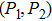in space is also analyzed. Discussion is presented in the last section.

## 2 Libration Model

Study of the rotation of the Moon is based on the following three laws provided by Cassini (Beletskii ):

The Moon rotates about its polar axis with constant angular velocity equal to that of its revolution around Earth.

The inclination of the Moonʼs equator with respect to the plane of the ecliptic is constant.

The poles of the Moonʼs axis of rotation, those of the ecliptic, and those of the lunar orbit, lie in one great circle.

When we use the selenocentric coordinate system, it is supposed that the Moon has the form of a triaxial ellipsoid, with its longest axis directed towards the mean direction of the Earth, and the smallest one is the axis of rotation. We direct OX 1 along the longest axis, OX 3 along the axis of rotation with positive being northwards and OX 2 so as to form a right-handed coordinate system. The directions of these axes with respect to the mean Earth equatorial system at J2000.0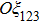are defined by three Euler angles Θ, Ψ and Φ, where Θ is the inclination of the lunar equator with respect to the mean Earth plane, Ψ is the angle along the mean Earth equatorial plane and Φ is the rotation angle along the lunar equatorial plane (see Figure 1). However, the three Euler angles mentioned above are not on the mean ecliptic of date, thus, we need to transform the Euler angles from the J2000.0 equatorial Earth reference system to the mean ecliptic of date reference system by applying four successive rotation matrices BPNR1(ε), where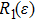denotes the rotation along the X-axis, and B, P and N are the frame bias, precession and nutation matrices, respectively. The four matrices can be calculated using SOFA routines (Hohenkerk ), then we will get the new Euler angles,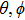and ψ.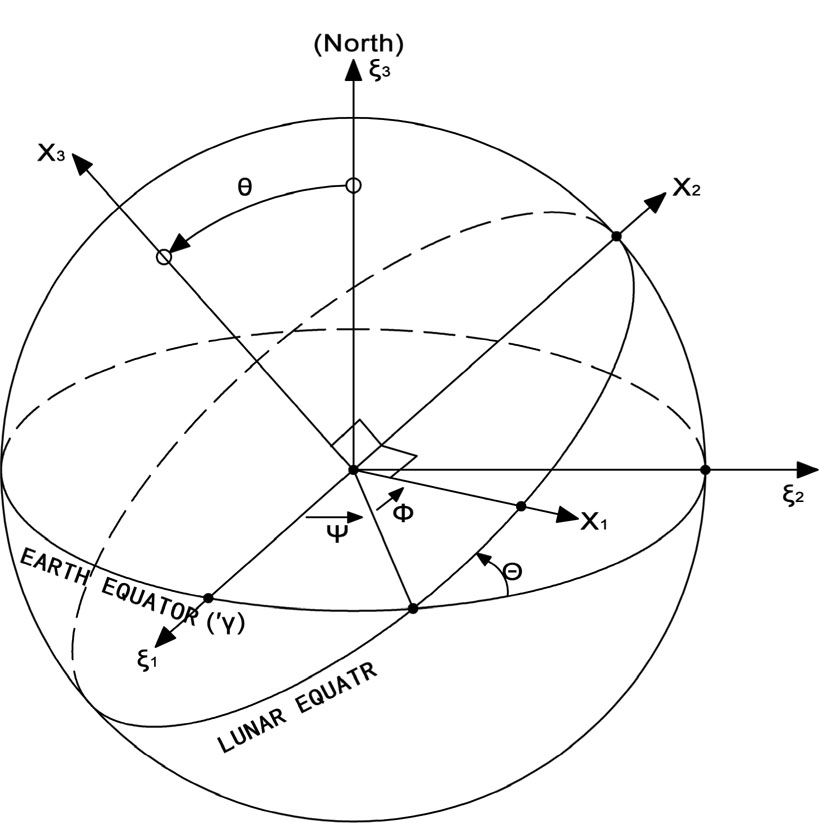Fig. 1. Euler angles used to describe the orientation of the selenocentric principal axis system (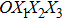) relative to the mean Earth equatorial system at J2000 (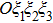).

If Cassiniʼs laws hold perfectly when applied, the Euler angles would satisfy the equations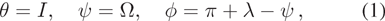where I is the mean inclination of the lunar equator with respect to the ecliptic and equal to about 1.543°, Ω is the longitude of ascending node of the lunar orbit and λ is the mean longitude of the Moon. The deviation of the angles θ, ψ and ϕ from the Cassini state,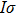, ρ and τ, are designated as the libration angles (Eckhardt ), where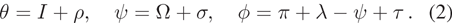Usually, σ is multiplied by I to be comparable to ρ, ρ and, which are referred to as the latitude librations and τ is referred to as the longitude libration. The pole position of the ecliptic of date on the lunar equator coordinate system is denoted by(Eckhardt ; Petrova ), which is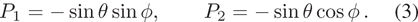To describe the pole oscillations around the equilibrium position, we use P 1,P 2 and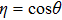as the unit vector to show the pole position in space.

Time variations of the libration angles and polar coordinates are shown in Figures. 2 and 3 for 1100 yr. We found that the width of the circle at the top and bottom is thinner than that at the side in the left panel of Figure 3. Although the difference is very small, we will discuss it in Section 5.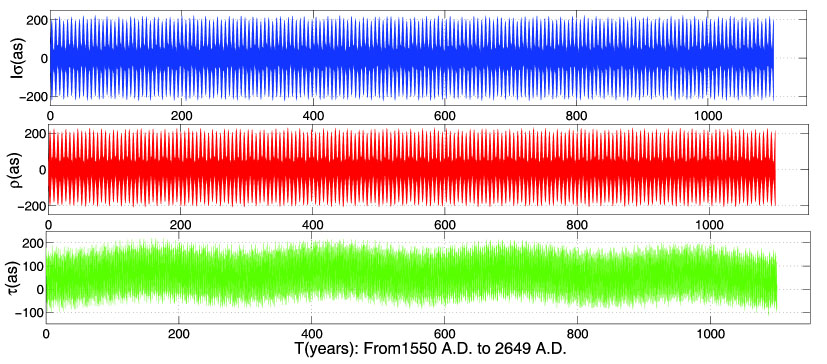Fig. 2. Temporal evolution of the three librations angles over 1100 yr.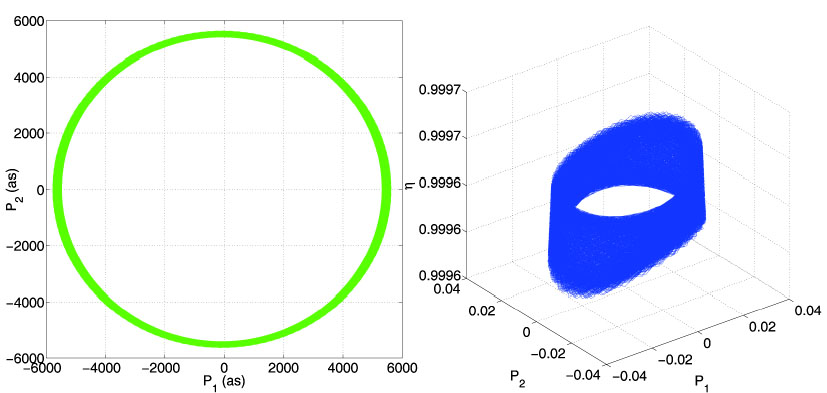Fig. 3. Ecliptic pole precessional cone over 1100 yr (left) and pole oscillation unit vector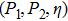in space (right).

## 3 Free libration of the Moon

The lunar physical librations have three components of free modes: two components of the pole, P 1 and P 2, and one longitudinal τ. In order to describe lunar rotation, the Moon is usually considered as a solid body, and the elastic deformation and presence of the core alter the proper periods by only small amounts (Williams et al. ). In this case, P 1,P 2 and τ which are functions of time can be determined from the well known Euler-Liouville equations,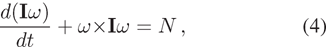where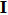is the inertia tensor, N is the total of all externally applied torques and ω is the rotation vector.

We get the linear approximation of the differential equations as Rambaux and Williams (), and add a small correction term (Moons ) to the third one,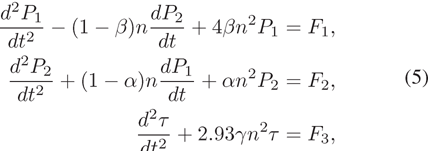where n is the lunar mean motion,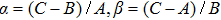and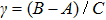, with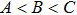the principal moments of inertia, and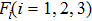are forcing functions. In this case, the eigen-frequencies of this linear system are corresponding to the proper period of lunar librations (Li et al. ).

The longitude frequency is obtained as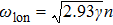, and the latitude and wobble are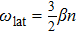and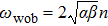(referring to an inertial system) respectively. Using the most recent data from GRAIL, we computed the eigen-frequencies of the free librations (Table 1).

Mode Eigen-frequency (rad d−1) Period (d) Period (yr)
Longitude 5.9404×10−3 1057.6976 2.8958
Latitude 2.1767×10−4 28865.0161 79.0281
Wobble 2.3202×10−4 27079.8675 74.1406
Table 1.

Frequencies and Periods of Free Librations from Theory.

## 4 Frequency analysis

The forced and free lunar physical librations are included together in the ephemeris. Firstly, we obtain the Euler angles from DE430 covering 1100 yr (from 1550 A.D. to 2649 A.D.), then we transform the angles from the equatorial reference frame with equinox at J2000.0 to the ecliptic of date. We use I=0.02692 radian and Ω, λ from Simon et al. . To estimate the components of the parameters, ρ, τ, P 1 and P 2, a fitting model is needed. Let us assume the solution of those five quantities has the form,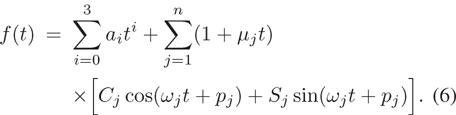To estimate the polynomial coefficients a i , Poisson term μ j , Fourier series coefficients C j , S j and phase p j by the least-squares method, we need to know the frequencies ω j precisely. We employ Fourier analysis that is part of the improved version and the estimation procedure as follows:

Step 1 Subtract the mean value, then use the Hanning window function.

Step 2 Find an approximate frequency with the maximum power by applying a Fast Fourier Transform (FFT). Then in the vicinity of the frequency, search for the maximum amplitude of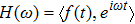with a quadratic interpolation method, where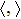means scalar product.

Step 3 This will give us the best frequency ω and then compute the projection of f(t). The contribution of the first frequency is then subtracted from f(t).

Step 4 Iterate this process until there are enough parameters.

In order to obtain the linear Poisson term μ j , we divide the data into subsets covering 300 yr and apply Fourier analysis to them. Then, by linear regression of the amplitudes as the first estimation, we determine whether the Poisson term is necessary or not. However, the disadvantage of this method is that any nonlinear term will influence the constant term. Then we apply the least-squares method to fit the form (6).

## 5 Determination of lunar free librations

The amplitude and phases of free librations can be determined from the fitting process. The wobble modeis the largest in the selenocentric reference system, and the semi-axes of the ellipse are obtained as, with respect to the polar axis. With the wobble period of 74.642 yr, P 1 and P 2 are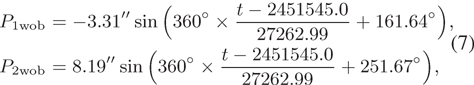where 2451545.0 is Julian date of the corresponding epoch, 2000 January 1, at 12h.

As for the latitude mode, thanks to the long term ephemeris series DE430 spanning 1100 yr, we can fit the small mode with an amplitude ofand a period of 24.112 yr (8806.9 d) when referring to the precession with the node, but the motion of the rotation axis will be retrograde with an 79.02 yr period as viewed from the inertial frame (Table 1).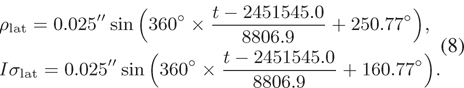We need to point out that the free libration in longitude is combined with two forced libration terms (Eckhardt ) arising from Venus at periods of 2.8923 yr and 2.8921 yr, and the mixed term has a period of 2.8917 yr (1056.207 d) with an amplitude of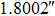, and a phase of 223.41° at epoch J2000.0 as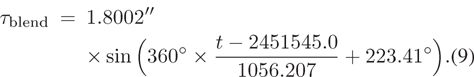In this case, we use Eckhardt’s theory to derive the two forced terms and then subtract them from the mixed term. By the least-squares method, we can get the amplitude and phase of the longitudinal free libration with the assumed period. The obtained free libration in longitude has a period of 1056.16 d, an amplitude ofand a phase of 210.5° at epoch J2000.0 as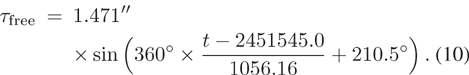Table 2 lists the periods, amplitudes and phases of the free librations that we found and also previous results from ephemeris DE421 (Rambaux & Williams ) and DE403 (Newhall & Williams ; Chapront et al. ).

Longitude blend Longitude mode Latitude mode Wobble mode
Period (d)
This work 1056.21 1056.16 8806.9 27262.99
Rambaux and Williams () 1056.21 1056.13 8822.88 27257.27
Newhall and Williams () 1056.20 1056.12 8826 27257.27
Chapront et al. () 1056.12 8804 27259.29
Amplitude (arc)
This work 1.8002 1.471 0.025 8.19 × 3.31
Rambaux and Williams () 1.8080 1.296 0.032 8.183 × 3.306
Newhall and Williams () 1.8070 1.37 0.022 8.19 × 3.31
Chapront et al. () 1.8120 0.022 8.182
Phase at JD 2451545 (°)
This work 223.41 210.5 250.67* 161.64
Rambaux and Williams () 223.5 207.0 160.8 161.60
Newhall and Williams () 223.8 208.9 246.4 161.82
Chapront (99) 224.3 250.3 161.77

Notes: The difference in phase between this paper and Rambaux and Williams () of the latitude mode is because we used the sine function to fit but they used the cosine function.

Table 2.

Free Librations Determined from Ephemeris DE430 and Comparison with the Previous Results.

The ephemerides DE421 and DE430 include a fluid core with an oblate core-mantle boundary. This will lead to the fourth free mode, i.e. free core nutation (FCN). The period of FCN, as given by Rambaux and Williams (), is 197 yr in DE421, but in DE430 the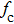(core oblateness) changes by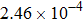and it becomes 300 yr. This difference, which comes from the period of the fourth mode, is proportional to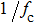, so a smallercauses a longer period.

As for P 1 and P 2, we find the second largest amplitude term has a period of 2190 d (5.99 yr) with different amplitudes of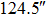and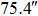. This leads to the unequal width of the circle in the left panel of Figure 3, and we can see it is consistent with the right panel.

## 6 Discussion

In this paper, based on the most accurate ephemeris DE430, we have determined the periods, amplitudes and phases of three free libration modes by FFT, which are part of the improved version, and the least-squares method. Comparisons with previous results are shown in Table 2, and they are similar but different in detail. We estimated the amplitudes asin longitude,in latitude andfor the wobble, with the respective periods of 1056.16, 8806.9 and 27262.99 d. Due to the different ephemeris using a different lunar interior model, the different ephemeris gives different values of amplitude and phase. On the other hand, the FCN frequency is very uncertain.

The amplitude of the free libration depends on excitation and damping processes. Free librations will eventually be damped to zero in the absence of excitation. The observational detection of free librations requires recent existence of excitation to counter damping. Without recent excitation, the free librations are therefore expected to be completely damped. Some possible excitation mechanisms have been proposed in the past, although they were not satisfactory. Passage through a resonance was proposed by Eckhardt (), and existence of a liquid core was proposed by Yoder ().

With the continuation of LLR, more and more distance data between the Moon and Earth have been accumulated. Moreover, during the extension of the Chinese Chang’e-3 lander mission, lunar microwave ranging technology with sub-millicycle accuracy (Ping et al. ) was realized and implemented from 2015 in China. The new determination of free librations modes will enable investigation of their mechanisms.

Acknowledgements The Planetary and Lunar Ephemer- ides DE430 were downloaded from the National Aeronautics and Space Administration (NASA). The coordinate transformations were performed using SOFA, which is available at http://www.iausofa.org. This research is supported by the National Natural Science Foundation of China (Grant No. 41590851) and the Major State Basic Research Development Program of China (2015CB857101).

 Beletskii V. V. 1972 Celest. Mech. 6 356 Calame O. 1977 Astrophysics and Space Science Library, 62, Scientific Applications of Lunar Laser Ranging Mulholland J. D. 53 Chapront J. Chapront-Touzé M. Francou G. 1999 A&A 343 624 Eckhardt D. H. 1965 AJ 70 466 Eckhardt D. H. 1981 Moon and Planets 25 3 Eckhardt D. H. 1982 Astrophysics and Space Science Library, 94, IAU Colloq. 63: High-Precision Earth Rotation and Earth-Moon Dynamics: Lunar Distances and Related Observations Calame O. 193 Eckhardt D. H. 1993 Celest. Mech. Dyn. Astron. 57 307 Folkner W. M. Williams J. G. Boggs D. H. 2009 Interplanetary Network Progress Report 178 1 Folkner W. M. Williams J. G. Boggs D. H. Park R. S. Kuchynka P. 2014 Interplanetary Network Progress Report 196 1 Hohenkerk C. 2011 Scholarpedia 6 11404 Jin W. Li J. 1996 Earth Moon and Planets 73 259 Li W. Gusev A. Ping J. Zhang T. 2014 Journal of Deep Space Exploration 1 205 (in Chinese) http://jdse.bit.edu.cn/sktcxbcn/ch/reader/view_abstract.aspx?file_no=201403008&flag=1 Moons M. 1982 Moon and Planets 27 257 Newhall X. X. Williams J. G. 1996 Celest. Mech. Dyn. Astron. 66 21 Petrova N. 1996 Earth Moon and Planets 73 71 Ping J. Li W. Han S. 2017 Scientia Sinica Physica, Mechanica & Astronomica 47 059508 Rambaux N. Williams J. G. 2011 Celest. Mech. Dyn. Astron. 109 85 Simon J. L. Bretagnon P. Chapront J. 1994 A&A 282 663 Standish E. M. 1995 BAAS, 27, Bulletin of the American Astronomical Society Butler B. J. Muhleman D. O. 1203 Williams J. G. Boggs D. H. Yoder C. F. Ratcliff J. T. Dickey J. O. 2001 J. Geophys. Res. 106 27933 Williams J. G. Konopliv A. S. Boggs D. H. 2014 Journal of Geophysical Research (Planets) 119 1546 Yoder C. F. 1981 Philosophical Transactions of the Royal Society of London Series A 303 327
Cite this article: Yang Yong-Zhang, Li Jin-Ling, Ping Jin-Song, Hanada Hideo. Determination of the free lunar libration modes from ephemeris DE430. Res. Astron. Astrophys. 2017; 12:127.

### Refbacks

• There are currently no refbacks.